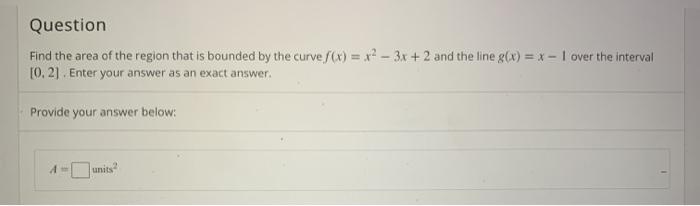# Question Solved1 AnswerQuestion Find the area of the region that is bounded by the curve f(x) = x2 – 3x + 2 and the line g(x) = x - 1 over the interval 10,2). Enter your answer as an exact answer. Provide your answer below:Transcribed Image Text: Question Find the area of the region that is bounded by the curve f(x) = x2 – 3x + 2 and the line g(x) = x - 1 over the interval 10,2). Enter your answer as an exact answer. Provide your answer below:
More
Transcribed Image Text: Question Find the area of the region that is bounded by the curve f(x) = x2 – 3x + 2 and the line g(x) = x - 1 over the interval 10,2). Enter your answer as an exact answer. Provide your answer below: